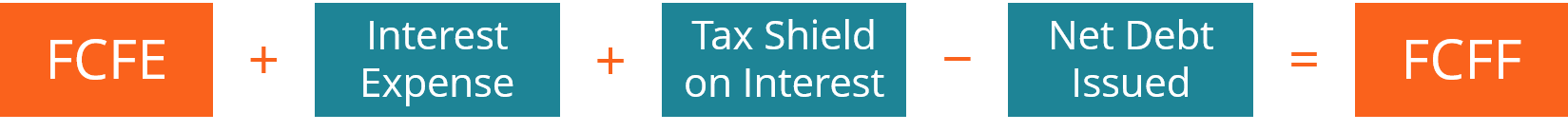# Forecasting Cash Flow

Part four of the four-step financial forecasting model in Excel

## Forecasting Cash Flow in a Financial Model

This article on forecasting cash flow is the last part of the four-step financial forecasting model in Excel. Having completed our income statement and balance sheet forecasts, we can now turn to the cash flow statement to complete the four-step forecast modeling framework.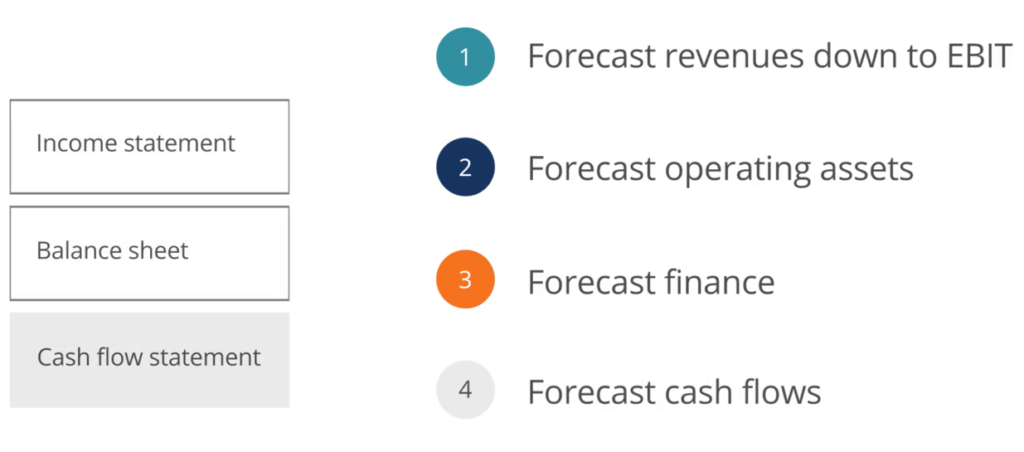• Understand the importance of incorporating error checks in forecasting cash flow
• Derive a forecast cash flow statement based on a forecast income statement or balance sheet
• Derive a free cash flow statement that can be used for equity valuation

### Forecasting Financial Statements

A cash flow forecast can be derived from the balance sheet and income statement. We begin by forecasting cash flows from operating activities before moving onto forecasting cash flows from investing and financing activities. Operating activities include revenues and operating expenses while investing activities include sale or purchase of assets and financing activities with the issuance of shares and raising debt. From forecasting all three activities, we will arrive at the forecast net cash movement.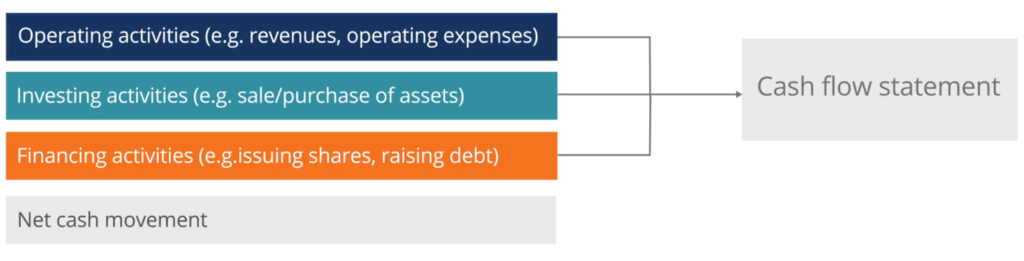### Cash Flows from Operating Activities

The first step in our cash flow forecast is to forecast cash flows from operating activities, which can be derived from the balance sheet and the income statement.

From the income statement, we use forecast net income and add back the forecast depreciation. We then use the forecast balance sheet to calculate changes in operating assets and liabilities. For each operating asset and liability, we must compare our forecast year in question with the prior year. In this example, changes in receivables and inventory have the effect of increasing the total cash flows. In other words, receivables and inventory in our forecast year are both lower than the prior year.

### Cash Flows from Investing Activities

Changes in trade and other payables have a reverse effect – decreasing total cash flows from operating activities. In other words, the payables figure must be lower in our forecast year than the prior year.

Now that we learned how to calculate cash flows from operating activities, let’s look at investing activities. All investing activity items come from specific fixed assets or property plant & equipment (PP&E) forecasts.

Our model forecasts fixed assets in detail in the “Supporting Schedules” section, where we assume:

• Assets are fully depreciated when disposed of and no cash flows are associated with the disposals
• No purchase or sale of businesses

As a result, the only item we will forecast in our model will relate to the acquisition of fixed assets or property plant & equipment (PP&E). It is often referred to as CAPEX, shortened for capital expenditures.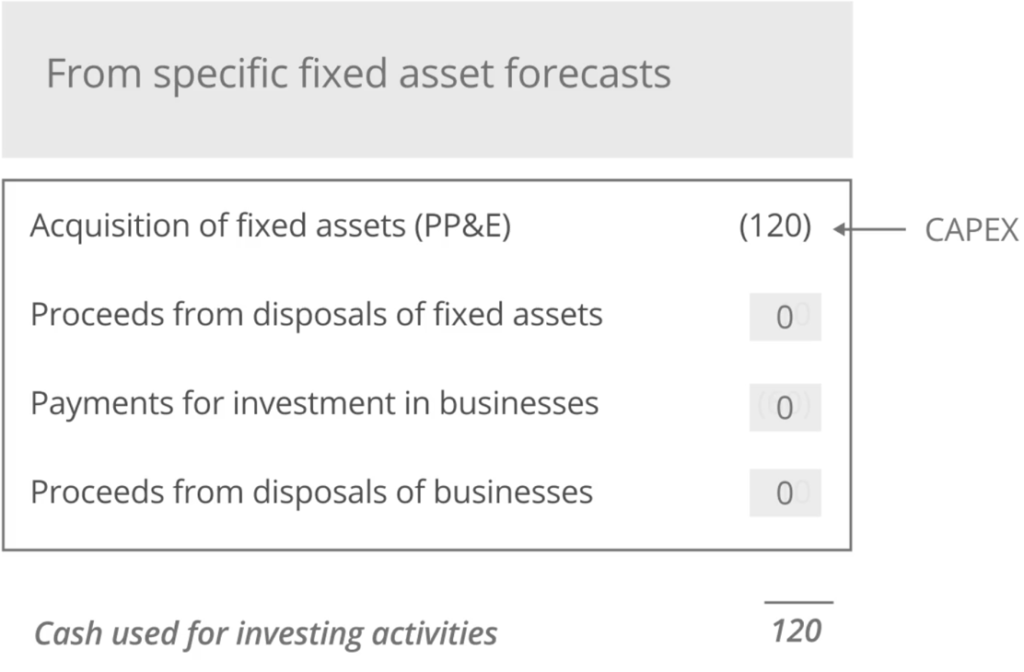### Cash Flows from Financing Activities

After forecasting investing activities, we will now learn how to calculate cash flows from financing activities. Most financing activity items are calculated by simply comparing the forecast year with the prior year. In our model, we included dividends as our financing activity. In practice, some organizations include dividend cash flows in operating activities. The choice should reflect how dividends are reported in financial statements.

### Forecasting Free Cash Flow

Free cash flow to the firm (aka Unlevered Free Cash Flow) forecast is the preferred approach when valuing equities using discounted cash flows. Free cash flows to the firm can be defined by the following formula: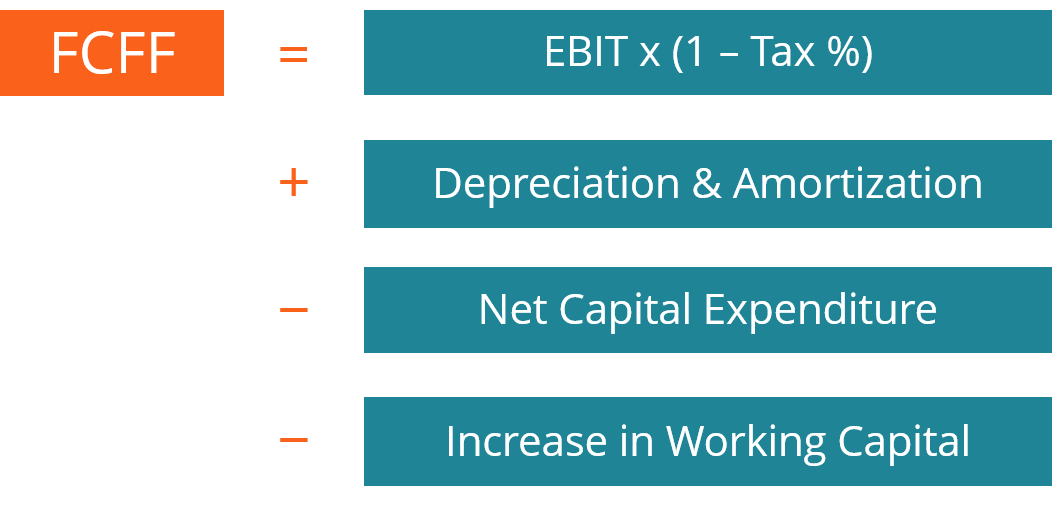FCF to the firm is Earnings Before Interests and Taxes (EBIT) times one minus the tax rate, where the tax rate is expressed as a percent or decimal. Since depreciation and amortization are non-cash expenses, they are added back. Net capital expenditures and increases in net working capital are then deducted. Note that decreases in working capital will be added to the equation.

### Forecasting Free Cash Flow to Equity

Although FCF to the firm is the preferred approach to equity valuation, it is not the only FCF calculation used. There is another FCF variant that is used called FCF to equity.

Free cash flows to equity are used to determine how much cash is available to equity investors after paying off debt interests and satisfying its sustainable obligations. In simple terms, FCF to equity is cash flow from operations minus capital expenditures plus net debt issued.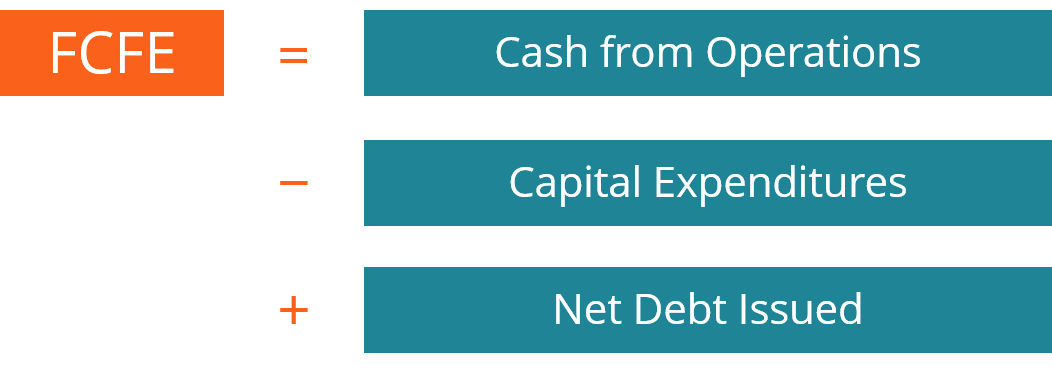### Reconciling Free Cash Flows

Since there are only two major differences between FCF to the firm and FCF to equity, it is relatively easy to reconcile the two.

Starting with FCF to equity, we simply deduct the net debt issued, add back the interest expense and deduct the tax shield on interest. The tax shield on interest is the difference between taxes calculated on EBIT and taxes calculated on earnings before tax.